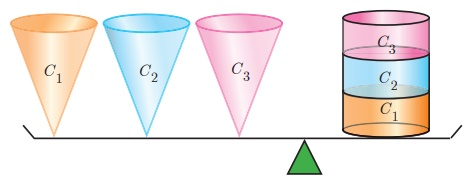Home | | Maths 10th Std | Volume of a right circular cone

# Volume of a right circular cone

Let r and h be the radius and height of a cone then its volume

Volume of a right circular cone

Let r and h be the radius and height of a cone then its volume

V = 1/3 × πr2h cu. units.

Demonstration

·        Consider a right circular cylinder and three right circular cones of same base radius and height as that of the cylinder.

·        The contents of three cones will exactly occupy the cylinder.From, Fig.7.28 we see that,

3× (Volume of a cone) = Volume of cylinder

= πr 2h cu. Units

Volume of a cone = 1/3 πr2h cu. Units

### Example 7.19

The volume of a solid right circular cone is 11088 cm3. If its height is 24 cm then find the radius of the cone.

### Solution

Let r and h be the radius and height of the cone respectively.

Given that, volume of the cone = 11088 cm3Therefore, radius of the cone r=21 cm

Example 7.20 The ratio of the volumes of two cones is 2:3. Find the ratio of their radii if the height of second cone is double the height of the first.

Solution Let r1 and h1 be the radius and height of the cone-I and let r2 and h2 be the radius and height of the cone-II.Therefore, ratio of their radii = 2 : √3

Tags : Definition, Formula, Solved Example Problems | Mensuration | Mathematics , 10th Mathematics : UNIT 7 : Mensuration
Study Material, Lecturing Notes, Assignment, Reference, Wiki description explanation, brief detail
10th Mathematics : UNIT 7 : Mensuration : Volume of a right circular cone | Definition, Formula, Solved Example Problems | Mensuration | Mathematics